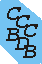Computational Chemistry Comparison and Benchmark DataBase Release 22 (May 2022) Standard Reference Database 101 National Institute of Standards and Technology Home All data for one species Geometry Experimental Calculated Comparisons Bad Calculations Tutorials and Explanations Vibrations Experimental Calculated Scale factors Reactions Entropies Ions List Ions Energy Electron Affinity Proton Affinity Ionization changes point group Experimental One molecule all properties One property a few molecules Geometry Vibrations Energy Electrostatics Reference Data Calculated Energy Optimized Reaction Internal Rotation Orbital Nuclear repulsion energy Correlation Ion Excited State Basis Set Extrapolation Geometry Vibrations Frequencies Zero point energy (ZPE) Scale Factors Bad Calculations Electrostatics Charges Dipole Quadrupole Polarizability Spin Entropy and Heat Capacity Reaction Lookup by property Comparisons Geometry Vibrations Energy Entropy Electrostatics Ion Resources Info on Results Calculations Done Basis functions used I/O files Glossary Conversion Forms Links NIST Links External links Thermochemistry Tutorials Vibrations Entropy Energy Electrostatics Geometry Cost Bad Calculations FAQ Help Units Choose Units Explanations Credits Just show me Summary Using List Recent molecules Molecules Geometry Vibrations Energy Similar molecules Ions, Dipoles, etc. Index of CCCBDB Feedback You are here: Resources > Tutorials > Energy > Getting enthalpy of formation

# How to get an enthalpy of formation from ab initio calculations

1. Choose a reaction with experimentally known enthaplies of formation for all species except the one of interest.
2. Calculate the energies for all species in the reaction at the same level of theory.
1. What level of theory should I use?
2. Optimize the geometries.
3. Calculate vibrational frequencies. (Why do we need the vibrational frequencies?)
4. (Optional) Calculate the electronic energy for all optimized geometries at some higher level of theory.
3. (Optional) Convert from 0K to 298.15K (or some other temperature).
4. Combine the various energies to get a reaction energy.
1. For each species we want the electronic energy plus the vibrational zero point energy.
2. The reaction energy is the sum of the product energies minus the sum of the reactant energies.

Use the enthalpy computation form, which has an example.
Using ab initio methods we can obtain ideal-gas enthalpies of formation, though not usually directly. The ab initio calculation provides us with an energy (in hartrees) for the process of separating the molecule into bare nuclei and electrons. For example if we are interested in the enthalpy of formation of ethene (C2H4 ) we can run a calculation, say HF/6-31G* and get and energy for the optimized structure of -78.031718 hartrees. This energy is for the (not very useful) reaction:
R1. C2H4 = 2C6+ + 4H+ + 16e-
If we knew the experimental enthalpy of formation of each species of the right side of this reaction we could obtain the enthalpy of formation of difluoromethane. However it is better to use a reaction that keeps the electrons and nuclei together. This helps cancel the systematic errors in the calculations.
Experimentally a reaction energy is measured. From this reaction energy and known enthalpies of formation of all but one of the species in the reaction, the enthalpy of formation of the unknown can be determined. For example if we measured the enthalpy of reaction for ethene reacting with oxygen to yield water and carbon dioxide.
R2. C2H4 + 3O2 = 2H2O(gas) + 2CO2(gas)
We find a reaction enthalpy of -1,325.1 kJ/mol. The relation between the reaction enthalpy and the individual enthalpies of formation is just ΔrH = Σ ΔfH(product) - Σ ΔfH(reactant). For this example:
Eq. 1 -1325.1 kJ/mol = 2 ΔfH(H2O) + 2 ΔfH(CO2) - ΔfH(C2H4) - 3 ΔfH(O2)
Plugging in the known enthalpies of formation of H2O, CO2 and O2 (The enthalpy of formation of O2 is defined to be 0 kJ/mol):
Eq. 2 -1325.1 kJ/mol = 2 (-238.9 ) + 2 (-393.1) - ΔfH(C2H4) - 3 ΔfH(0)
Rearranging this equation yields the enthalpy of formation of ethene ΔfH(C2H4) = 61.1 kJ/mol.
We use a similar a similar procedure for determining the enthalpy of formation of a species using ab initio calculations. We get the energy of the reaction by calculating the ab initio energy of each species. Using HF/6-31G* calculated energies we have:
 Species electronic energy(hartrees) vibrational zpe (cm-1) total energy (hartrees) C2H4 -78.031718 10763 -77.982677 O2 -149.617908 895 -149.613830 H2O -76.010747 4515 -75.990175 CO2 -187.634176 2505 -187.622762

The reaction energy is 2 (-75.990175) + 2 (-187.622762) - (-77.982677) - 3 (-149.613830)
or -0.401706 hartrees (-1054.66 kJ/mol).

Using this reaction enthalpy and the experimental values for the enthalpy of formation of H2O, CO2 and O2 we have, similar to Eq. 2 above:
Eq. 3 -1054.66 kJ/mol = 2 (-238.9 ) + 2 (-393.1) - ΔfH(C2H4) - 3 ΔfH(0)
This yields the enthalpy of formation of ethene ΔfH(C2H4) = -209.34 kJ/mol. This poor result (we're off by 270 kJ/mol!) can be improved by the choice of a better reaction. Ideally an isodesmic reaction (one that conserves bond types) is best, but even an isogyric reaction (one that conserves spin - O2 is a triplet species) helps to cancel out systematic errors in the ab initio calculations.
An isodesmic reaction involving ethene could be between ethene and propane to yield ethane and propene. The number of each bond tpye is conserved: 1 C=C bond, 2 C-C bonds, 12 C-H bonds.
R3. C2H4 + C3H8 = C2H6 + C3H6
Using energies from an HF/6-31G* calculation we obtain a reaction energy of -13.1 kJ/mol. Using experimental values for the heat of formation of C3H8(-82.40), C2H6(-68.38), and C3H6(34.70) we can obtain the enthalpy of formation of ethene of 61.82 kJ/mol. This value is much closer to the reported experimental value of 60.99 kJ/mol.

In all the above examples we have used experimental enthalpies of formation reported at 0 K (zero Kelvin). Usually it is more useful to predict enthalpies of formation at room temperature (298.15 K) or some other temperature. To do this we need to convert our 0 K enthalpy of formation to an enthalpy of formation at our desired temperature. This is described on the next page.